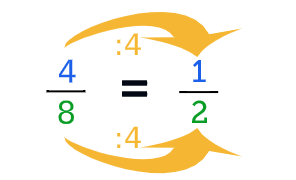# Simplifying Fractions Level 1

## The 5-step planHere you can learn to simplify fractions with the 5-step plan. When you simplify a fraction, you make a fraction a small as possible. Divide the fraction by the greatest common factor. This way you get the simplest form of a fraction, with the smallest possible numerator an denominator. The 5 steps of the 5-step plan are as follows: At step 1 you only need to fill in the numerator (top number). At step 2 you drag the answer to the matching question. At step 3 you need to fill in the numerator and denominator (bottom number). At step 4 there are 15 multiple choice questions. And finally step 5, the diploma with 20 questions.

### Step 4 - Multiple choice

Try to answer all the 15 questions right!

### Step 5: Get your diploma

Answer all the 20 questions right to get the diploma!

## Description Simplifying Fractions 1

When you want to simplify fractions, you need to look for the greatest common factor, also called the GCF. This is the highest positive cardinal number both the numerator and denominator can be divided by, without leaving remainders. The greatest common factor of the numbers 6 and 9, for example, is 3.